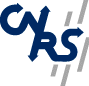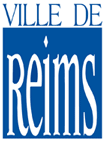Franco-British "alliance" workshop and Conference in honour of Jacques Alev's sixtieth birthday 27-28-29 November 2008 University of Reims Main page Program Participants Titles and abstracts Inscription Practical informations Version française G. Bellamy (Edinburgh Univ.): Factorization in generalized Calogero-Moser spaces In this talk I shall describe some of the properties of the centres of rational Cherednik algFactorization in generalized Calogero-Moser spacesebras at "t = 0". In particular, I shall show that they satisfy a certain "factorization" property, and discuss the consequences of this for the representation theory of these algebras. K. Brown (Glasgow Univ.): Small infinite non-commutative groups I'll review recent work of myself, Goodearl and Zhang on the structure and classification of Hopf algebras of small Gelfand-Kirillov dimension. A. Joseph (Weizmann Institute): A Pentagonal Crystal and the Golden Section Ch. Kassel (Strasbourg 1 Univ.): Homology groups for Hopf algebras In the 1960's Sweedler introduced a cohomology theory for cocommutative Hopf algebras, generalizing both group and Lie algebra cohomology. Sweedler's 2-cocycles are essential for the construction of Hopf Galois extensions. They make sense even for non-cocommutative Hopf algebras. Nevertheless in the general case, 2-cocycles no longer form a group, which makes it difficult to build a cohomology group out of them. A few years ago, Bichon, Carnovale, Chen, Schauenburg et al. came up with the idea of restricting to a class of special 2-cocycles, called the lazy cocycles. Using the latter they built a group of lazy 1-cohomology and a group of lazy 2-cohomology. When the Hopf algebra is cocommutative, these groups coincide with the corresponding Sweedler cohomology groups.In my talk I'll report about recent joint work with Julien Bichon (Clermont-Ferrand), in which we build two bicommutative Hopf algebras H_1(H) and H_2(H) for each Hopf algebra H. These algebras play the role of degree 1 and 2 homology groups; they are related to the lazy 1-cohomology and 2-cohomology groups by universal coefficient theorems. When H is a group algebra, then H_i(H) can be expressed in terms of the 1- and 2-homology of the group. When H is a cosemisimple Hopf algebra over an algebraically closed field of characteristic zero (e.g., when H is the Hopf algebra of functions on a finite group), then H_1(H) is the Hopf algebra of the universal grading group of the category of corepresentations of H. We also computed H_1(H) et H_2(H) in the case H is Sweedler's four-dimensional Hopf algebra. B. Keller (Paris 7 Univ.): The periodicity conjecture via 2-Calabi-Yau categories The periodicity conjecture was formulated in mathematical physics at the beginning of the 1990s, in the work of Zamolodchikov, Kuniba-Nakanishi and Gliozzi-Tateo. It asserts that a certain discrete dynamical system associated with a pair of Dynkin diagrams is periodic and that its period divides the double of the sum of the Coxeter numbers of the two diagrams. The conjecture was proved by Frenkel-Szenes and Gliozzi-Tateo for the pairs (A_n, A_1), by Fomin-Zelevinsky in the case where one of the diagrams is A_1 and by Volkov and independently Szenes when both diagrams are of type A. The conjecture is about to be proved by Hernandez-Leclerc in the case where one of the diagrams is of type A. We will sketch a proof of the general case which is based on Fomin-Zelevinsky's work on cluster algebras and on the theory relating cluster algebras to triangulated 2-Calabi-Yau categories. An important role is played by Coxeter transformations and by Amiot's recent work on cluster categories for algebras of global dimension 2. S. Launois (Kent Univ.) : Combinatorics in quantum matrices In this talk, we present combinatorial tools in order to study the representation theory of the algebra of quantum matrices. This is joint work with Jason Bell. L. Le Bruyn (Antwerp Univ.): "Dessins d'enfant" I will try to convince you that Grothendieck's 'dessins d'enfant' form an example of a noncommutative manifold over the mythical field with one element (in the sense of Soule and Connes-Consani). B. Leclerc (Caen Univ.): Canonical and semicanonical bases : an example Lusztig and Kashiwara have defined canonical bases, via quantum enveloping algebras. Lusztig also defined semicanonical bases of (classical) enveloping algebras. On the other hand, Sherman and Zelevinsky have introduced canonical bases for certain cluster algebras, characterized by some positivity properties. Caldero and Zelevinsky have also introduced semicanonical bases for the same cluster algebras. We shall present an example in which all these bases can be considered, and try to compare them. J.T. Stafford (Manchester Univ.) : Noncommutative projective surfaces This is joint work with Thierry Levasseur. The differential operators on a semisimple Lie algebra invariant under the action of the associated Lie group are fairly well-understood. The analogous invariant differential operators on symmetric spaces are less well-understood and certainly less well behaved. In this talk we will show that these algebras are closely related to Cherednik algebras and discuss some of the applications. R. Taillefer (Saint-Etienne Univ.): Coxeter polynomial of a finite-dimensional algebra with finite global dimension Let A be a finite dimensional algebra over an algebraically closed field. Assume A is basic and has finite global dimension. Let C_A be its Cartan matrix and let Phi_A=-C_A^{-t}C_A be its Coxeter matrix. In 1997, D. Happel gave a homological formula for the trace of Phi_A. Among other consequences of this result, he gave a combinatorial criterion to distinguish most piecewise hereditary algebras. However, for two different types of piecewise hereditary algebras, the trace of the Coxeter matrix is -1. M.J. Redondo, M. Lanzilotta and myself considered the other coefficients of the Coxeter polynomial, that is, the characteristic polynomial of the Coxeter matrix. We gave a homological interpretation of these coefficients and obtained combinatorial criteria to distinguish most, but not all, types of piecewise hereditary algebras using their Coxeter polynomial, and proved that the Coxeter polynomial does not distinguish the remaining cases. P. Tauvel (Poitiers Univ.): Algèbres de Lie résolubles et algèbres de Poisson Soient g une algèbre de Lie résoluble, S(g) son algèbre symétrique, Q un idéal premier g-invariant de S(g), et E l'ensemble des semi-invariants non nul de S(g)/Q. On étudie la structure de l'algèbre de Poisson (S(g)/Q)_E. M. van den Bergh (Hasselt Univ.): Non-commutative quadrics In this talk we discuss some abelian categories of which it can be argued that they are the correct non-commutative analogues of P^1xP^1.